# RLC parallel circuit with V=32V, R=1.5kohms, XL=3.6koms, & XC=6.5kohms.FInd IT & Z in rectangular and polar form.

Question
46 views

RLC parallel circuit with V=32V, R=1.5kohms, XL=3.6koms, & XC=6.5kohms.

FInd IT & Z in rectangular and polar form.

check_circle

examination of the given circuit

The given circuit is RLC parallel circuit having the value of the components as:

R=1.5kohms.

XL=3.6koms.

XC=6.5kohms.

Calculation of the frequency.

the impedance of the parallel RLC circuit is given as: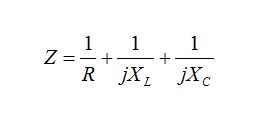Value of the XL=3.6koms.

Value of the XC=6.5kohms.

inductive resistance is given by the formula as shown below: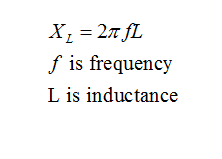similarily capacitive resistance is given by the formula as shown below: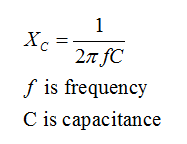calculation of the current

the value of the current is calculated by the value of the voltage and the magnitude of the impedance,

the value of the impedance magnitude  is calculated as: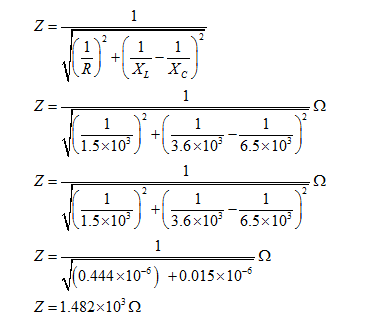The current is given as the ratio of the voltage to the impedance.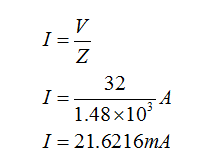...

### Want to see the full answer?

See Solution

#### Want to see this answer and more?

Solutions are written by subject experts who are available 24/7. Questions are typically answered within 1 hour.*

See Solution
*Response times may vary by subject and question.
Tagged in